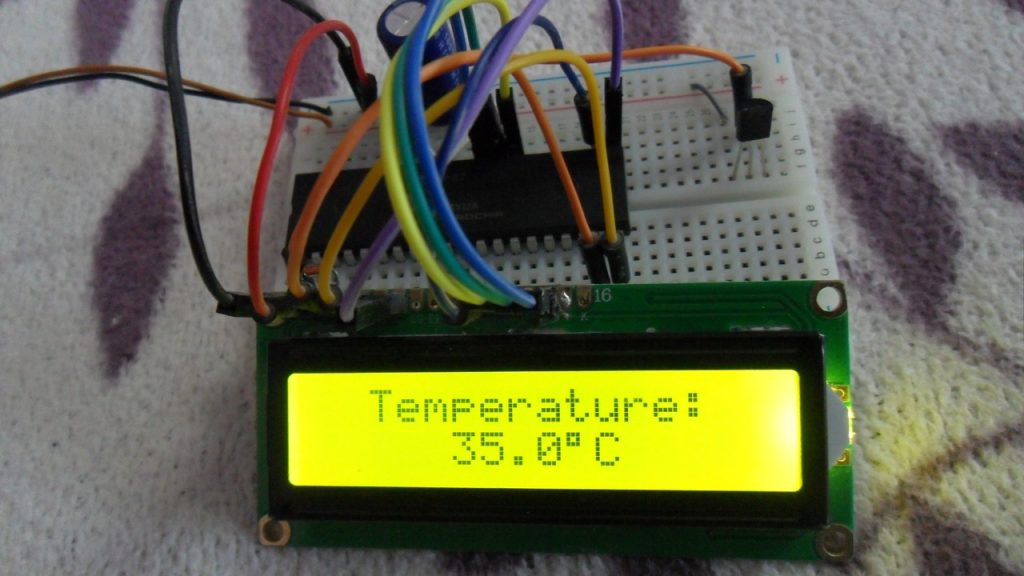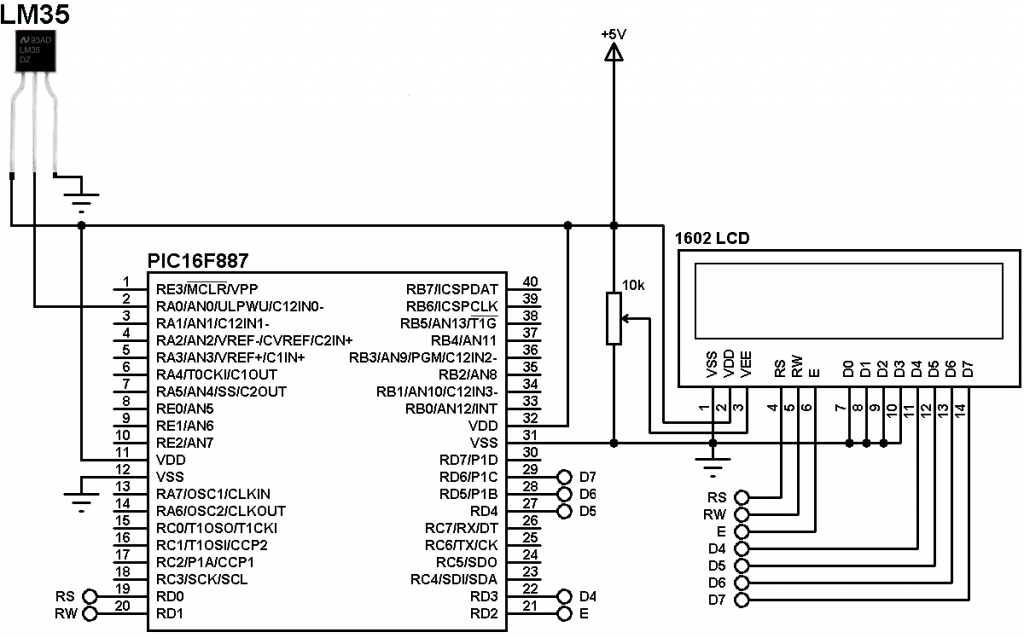# Interfacing PIC16F887 with LM35 temperature sensor – CCS C

A thermometer can easily be implemented using the low cost analog temperature sensor LM35. The LM35 temperature sensor is three pin device (VCC, OUT and GND) with an output voltage linearly related to Centigrade temperature. Since the LM35 output varies with dependent to the temperature we need ADC (Analog-to-Digital Converter) module to measure this voltage. The ADC module converts analog data into digital data.

The LM35 output has linear +10mV/°C scale factor means the following:
If the output voltage =   10mV —> temperature =   1°C
If the output voltage = 100mV —> temperature = 10°C
If the output voltage = 200mV —> temperature = 20°C
If the output voltage = 370mV —> temperature = 37°C
and so on.
This article shows the interfacing of the LM35 temperature sensor with PIC16F887 microcontroller.
The PIC16F887 microcontroller has one 10-bit ADC module with up to 14 channels. In this example one channel for the LM35 output is needed.

Hardware Required:

• PIC16F887 microcontroller
• LM35 temperature sensor  — datasheet
• 16×2 LCD screen
• 10K ohm variable resistor
• 5V voltage source
• Jumper wiresInterfacing PIC16F887 with LM35 temperature sensor circuit:In this project the PIC16F887 microcontroller uses its internal oscillator which is set in the C code and MCLR pin function is disabled.

Interfacing PIC16F887 with LM35 temperature sensor CCS C code:
Reading voltage quantity using the ADC gives us a number between 0 and 1023 (10-bit resolution), 0V is represented by 0 and 5V is represented by 1023. Converting back the ADC digital value is easy and we can use the following equation for that conversion: